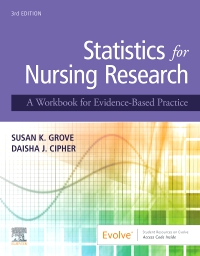ISBN: 9780323654111
Page Count: 496
Imprint: Elsevier
List Price: \$65.99

# Statistics for Nursing Research, 3rd Edition

by Susan K. Grove, PhD, RN, ANP-BC, GNP-BC and Daisha J. Cipher, PhD

## PaperbackISBN: 9780323654111
Page Count: 496
Imprint: Elsevier
List Price: \$65.99
Was \$65.99

### Now \$52.79

Free Shipping!
In Stock

Learn how to expand your interpretation and application of statistical methods used in nursing and health sciences research articles with Statistics for Nursing Research: A Workbook for Evidence-Based Practice, 3rd Edition. Perfect for those seeking to more effectively build an evidence-based practice, this collection of practical exercises guides you in how to critically appraise sampling and measurement techniques, evaluate results, and conduct a power analysis for a study. Written by nursing research and statistics experts Drs. Susan K. Grove and Daisha Cipher, this is the only statistics workbook for nurses to include research examples from both nursing and the broader health sciences literature. This new third edition features new research article excerpts and examples, an enhanced focused on statistical methods commonly used in DNP projects, new examples from quality improvement projects, new content on paired samples analysis, expanded coverage of calculating descriptive statistics, an online Research Article Library, and more! Whether used in undergraduate, master’s, or doctoral education or in clinical practice, this workbook is an indispensable resource for any nursing student or practicing nurse needing to interpret or apply statistical data.

• Comprehensive coverage and extensive exercise practice address all common techniques of sampling, measurement, and statistical analysis that you are likely to see in nursing and health sciences literature.
• Literature-based approach uses key excerpts from published studies to reinforce learning through practical application.
• 36 sampling, measurement, and statistical analysis exercises provide a practical review of both basic and advanced statistical techniques.
• Study Questions in each chapter help you apply concepts to an actual literature appraisal.
• Questions to Be Graded sections in each chapter help assess your mastery of key statistical techniques.
• Consistent format for all chapters enhances learning and enables quick review.
• NEW! Updated research articles and examples are incorporated throughout to ensure currency and relevance to practice.
• NEW! Enhanced focus on statistical methods commonly used in DNP projects and expanded coverage on calculating descriptive statistics broadens your exposure to the statistical methods you will encounter in evidence-based practice projects and in the literature.
• NEW! Examples from quality improvement projects provide a solid foundation for meaningful, high-quality evidence-based practice projects.
• NEW! Research Article Library on Evolve provides full-text access to key articles used in the book.
• NEW! Content on paired samples analysis familiarizes you with this type of research analysis.
• Part 1: Understanding Statistical Methods 1. Identifying Levels of Measurement: Nominal, Ordinal, Interval, and Ratio 2. Identifying Probability and Nonprobability Sampling Methods in Studies 3. Understanding the Sampling Section of a Research Report: Population, Sampling Criteria, Sample Size, Refusal Rate, and Attrition Rate 4. Understanding Reliability of Measurement Methods 5. Understanding Validity of Measurement Methods 6. Understanding Frequencies and Percentages 7. Interpreting Line Graphs 8. Measures of Central Tendency: Mean, Median, and Mode 9. Measures of Dispersion: Range and Standard Deviation 10. Description of a Study Sample 11. Interpreting Scatterplots 12. Algorithm for Determining the Appropriateness of Inferential Statistical Techniques 13. Understanding Pearson Product-Moment Correlation Coefficient 14. Understanding Simple Linear Regression 15. Understanding Multiple Linear Regression 16. Understanding Independent Samples t-test 17. Understanding Paired or Dependent Samples t-test 18. Understanding Analysis of Variance (ANOVA) and Post Hoc Analyses 19. Understanding Pearson Chi Square 20. Understanding Spearman Rank-Order Correlation Coefficient 21. Understanding Mann-Whitney U Test 22. Understanding Wilcoxon Signed-Rank Test

Part 2: Conducting and Interpreting Statistical Analyses 23. Selecting Appropriate Analysis Techniques for Studies 24. Describing the Elements of Power Analysis: Power, Effect Size, Alpha, and Sample Size 25. Conducting Power Analysis 26. Determining the Normality of a Distribution 27. Calculating Descriptive Statistics 28. Calculating Pearson Product-Moment Correlation Coefficient 29. Calculating Simple Linear Regression 30. Calculating Multiple Linear Regression 31. Calculating t-tests for Independent Samples 32. Calculating t-tests for Paired (Dependent) Samples 33. Calculating Analysis of Variance (ANOVA) and Post Hoc Analyses Following ANOVA 34. Calculating Sensitivity and Specificity 35. Calculating Pearson Chi-Square 36. Calculating Odds Ratio and 95% Confidence Intervals

References

Appendices Appendix A: Critical Values for Student's t Distribution Appendix B: Critical Values of r for Pearson Product Moment Correlation Coefficient Appendix C: Critical Values of F for a = 0.05 and a = 0.01 Appendix D: Critical Values of the ?2 Distribution

Index

• Susan K. Grove, PhD, RN, ANP-BC, GNP-BC, Professor Emeritus, College of Nursing and Health Innovation, The University of Texas at Arlington, Arlington, Texas; Adult Nurse Practitioner, Grand Prairie, Texas and Daisha J. Cipher, PhD, Associate Professor, College of Nursing and Health Innovation, The University of Texas at Arlington, Arlington, Texas
Was \$65.99

Free Shipping!
In Stock